# Analysis of the new homotopy perturbation method for linear and nonlinear problems

## Abstract

In this article, a new homotopy technique is presented for the mathematical analysis of finding the solution of a first-order inhomogeneous partial differential equation (PDE) ${u}_{x}\left(x,y\right)+a\left(x,y\right){u}_{y}\left(x,y\right)+b\left(x,y\right)g\left(u\right)=f\left(x,y\right)$. The homotopy perturbation method (HPM) and the decomposition of a source function are used together to develop this new technique. The homotopy constructed in this technique is based on the decomposition of a source function. Various decompositions of source functions lead to various homotopies. Using the fact that the decomposition of a source function affects the convergence of a solution leads us to development of a new method for the decomposition of a source function to accelerate the convergence of a solution. The purpose of this study is to show that constructing the proper homotopy by decomposing $f\left(x,y\right)$ in a correct way determines the solution with less computational work than using the existing approach while supplying quantitatively reliable results. Moreover, this method can be generalized to all inhomogeneous PDE problems.

## 1 Introduction

Many problems in the fields of physics, engineering and biology are modeled by linear and nonlinear PDEs. In recent years, the homotopy perturbation method (HPM) has been employed to solve these PDEs . HPM has been used extensively to solve nonlinear boundary and initial value problems. Therefore HPM is of great interest to many researchers and scientists. HPM, first presented by Ji Huan He , is a powerful mathematical tool to investigate a wide variety of problems arising in different fields. It is obtained by successfully coupling homotopy theory in topology with perturbation theory. In HPM, a complicated problem under study is continuously deformed into a simple problem which is easy to solve to obtain an analytic or approximate solution .

In this work, a new homotopy perturbation technique is proposed to find the solution $u\left(x,y\right)$ based on the decomposition of a right-hand side function $f\left(x,y\right)$ which leads to the construction of new homotopies. It is shown that decomposing $f\left(x,y\right)$ in a correct way, requiring less computational work than the existing approach, helps us to determine the solution. The results reveal that the proposed method is very effective and simple.

The paper is organized as follows. In Section 2, an analysis of the new homotopy perturbation method is given. The construction of a new homotopy based on the decomposition of a source function for a first-order inhomogeneous PDE is covered in Section 3. Some examples are given to illustrate the construction of a new homotopy in Section 4. Finally, some concluding remarks are given in Section 5.

## 2 New homotopy perturbation method

To illustrate the basic ideas of the homotopy analysis method, we consider the following nonlinear differential equation:

$A\left(u\right)-f\left(r\right)=0,\phantom{\rule{1em}{0ex}}r\in \mathrm{\Omega }$
(1)

with the boundary conditions

$B\left(u,\frac{\partial u}{\partial n}\right)=0,\phantom{\rule{1em}{0ex}}r\in \mathrm{\Gamma },$
(2)

where A is a general differential operator, B is a boundary operator, $f\left(r\right)$ is a known analytical function, and Γ is the boundary of domain Ω.

The operator A in (1) can be rewritten as a sum of L and N, where L and N are linear and nonlinear parts of A, respectively, as follows:

$L\left(u\right)+N\left(u\right)-f\left(r\right)=0.$

By the homotopy technique, we construct the homotopy

$H\left(v,p\right)=\left(1-p\right)\left(L\left(v\right)-L\left({u}_{0}\right)\right)+p\left(A\left(v\right)-f\left(r\right)\right)=0,$
(3)

which is equivalent to

$H\left(v,p\right)=L\left(v\right)-L\left({u}_{0}\right)+pL\left({u}_{0}\right)+p\left(N\left(v\right)-f\left(r\right)\right)=0,$
(4)

where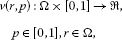p is an embedding parameter, ${u}_{0}$ is an initial approximation of (1), which satisfies the boundary conditions. As p changes from zero to unity, $v\left(r,p\right)$ changes from ${u}_{0}$ to $u\left(r\right)$. In this technique, the convergence of a solution depends on the choice of ${u}_{0}$, that is, we can have different approximate solutions for different ${u}_{0}$.

Let us decompose the source function as $f\left(r\right)={f}_{1}\left(r\right)+{f}_{2}\left(r\right)$. If we take

$L\left({u}_{0}\right)={f}_{1}\left(r\right)$

in (3), we obtain the following homotopy:

$H\left(v,p\right)=\left(1-p\right)\left(L\left(v\right)-{f}_{1}\left(r\right)\right)+p\left(A\left(v\right)-f\left(r\right)\right)=0,$
(5)

which is equivalent to

$H\left(v,p\right)=L\left(v\right)-{f}_{1}\left(r\right)+p\left(N\left(v\right)-{f}_{2}\left(r\right)\right)=0.$
(6)

Obviously, from (6) we have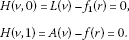As the embedding parameter p changes from zero to unity, $v\left(r,p\right)$ changes from ${L}^{-1}\left({f}_{1}\left(r\right)\right)$ to $u\left(r\right)$.

According to He’s homotopy perturbation method, we can first use the embedding parameter p as a small parameter and assume that the solution of (6) can be written as a power series in p as follows:

$v={v}_{0}+p{v}_{1}+{p}^{2}{v}_{2}+{p}^{3}{v}_{3}+\cdots .$
(7)

Setting $p=1$, we get the approximate solution of (1)

$u=\underset{p\to 1}{lim}v={v}_{0}+{v}_{1}+{v}_{2}+{v}_{3}+\cdots .$

In the next section, it is shown that the decomposition of the right-hand side function has a great effect on the amount of calculation and the speed of convergence of the solution.

## 3 Construction of a new homotopy based on the decomposition of a source function

Let us consider the following boundary value problem with the following inhomogeneous PDE: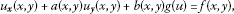(8)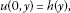(9)

where a, b, g and f are continuous functions in some region of the plane and $g\left(0\right)=0$. By solving this boundary value problem by the homotopy perturbation method, we obtain an approximate or exact solution $u\left(x,y\right)$. Before proceeding further, let us introduce the integral operator S defined in the following form:

$S\left(\cdot \right)={\int }_{0}^{x}\left(\cdot \right)\phantom{\rule{0.2em}{0ex}}dx.$
(10)

Then the derivative of operator S with respect to y is defined as

${S}_{y}\left(\cdot \right)=\frac{\partial }{\partial y}{\int }_{0}^{x}\left(\cdot \right)\phantom{\rule{0.2em}{0ex}}dx.$

Rewriting $f\left(x,y\right)$ as a sum of two functions $f\left(x,y\right)={f}_{1}\left(x,y\right)+{f}_{2}\left(x,y\right)$ and then constructing a homotopy in equation (6), we have

$H\left(v,p\right)=\left(1-p\right)\left({v}_{x}-{f}_{1}\right)+p\left({v}_{x}+a\left(x,y\right){v}_{y}+b\left(x,y\right)g\left(v\right)-f\right),$
(11)

which is equivalent to

$H\left(v,p\right)={v}_{x}\left(x,y\right)-{f}_{1}\left(x,y\right)+p\left(a\left(x,y\right){v}_{y}\left(x,y\right)+b\left(x,y\right)g\left(v\left(x,y\right)\right)-{f}_{2}\left(x,y\right)\right)=0.$
(12)

Substituting (7) into (12), and equating the coefficient of the terms by the same power in p, we have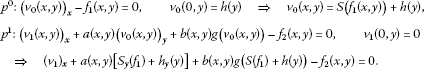(13)

If there exists the relationship

$a\left(x,y\right)\left[{S}_{y}\left({f}_{1}\left(x,y\right)\right)+{h}_{y}\left(y\right)\right]+b\left(x,y\right)g\left(S\left({f}_{1}\left(x,y\right)\right)+h\left(y\right)\right)={f}_{2}\left(x,y\right)$
(14)

between ${f}_{1}\left(x,y\right)$ and ${f}_{2}\left(x,y\right)$, then we have from (13)

${\left({v}_{1}\right)}_{x}=0,\phantom{\rule{2em}{0ex}}{v}_{1}\left(0,y\right)=0\phantom{\rule{1em}{0ex}}⇒\phantom{\rule{1em}{0ex}}{v}_{1}=0$

and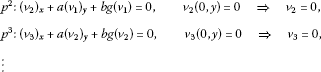Hence the approximate or exact solution of problem (8)-(9) is obtained as

$u\left(x,y\right)={v}_{0}\left(x,y\right),$

and the source function is obtained from (14) in the following form:

$f\left(x,y\right)={f}_{1}+a\left(x,y\right)\left[{S}_{y}\left({f}_{1}\right)+{h}_{y}\left(y\right)\right]+b\left(x,y\right)g\left(S\left({f}_{1}\right)+h\left(y\right)\right).$
(15)

Therefore equation (15) is a necessary condition to accelerate the convergence. If equation (15) has a solution, then we obtain the approximate or exact solution of problem (8)-(9) in two steps.

However, it is not always possible to decompose the source function $f\left(x,y\right)$ in such a way that the functions ${f}_{1}\left(x,y\right)$ and ${f}_{2}\left(x,y\right)$ have the relationship in (14). If we have such a case, then we are looking for an arbitrary $P\left(x,y\right)$ such that the functions ${f}_{1}\left(x,y\right)$ and ${f}_{2}\left(x,y\right)$ have the following relationship:

$a\left(x,y\right)\left[{S}_{y}\left({f}_{1}\right)+{h}_{y}\left(y\right)\right]+b\left(x,y\right)g\left(S\left({f}_{1}\right)+h\left(y\right)\right)={f}_{2}+P\left(x,y\right).$
(16)

In this case, we get the approximate or exact solution of the problem in more than two steps. Moreover, we can get the solution in the form of series.

## 4 Analysis of the new homotopy perturbation method for linear problems

In this section we illustrate the theory of the new homotopy perturbation method given in Sections 2 and 3 for some special $f\left(x,y\right)$ in the following linear problem: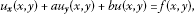(17)(18)

where a and b are constants.

### 4.1 Case 1: the source function $f\left(x,y\right)$ is a polynomial

We consider that $h\left(y\right)=0$ and $f\left(x,y\right)$ is an n th-order polynomial in problem (17)-(18). Hence $f\left(x,y\right)$ can be written in the following form:

$f\left(x,y\right)=\sum _{k=0}^{n}\sum _{\alpha +\beta =k}{A}_{\alpha \beta }{x}^{\alpha }{y}^{\beta },$
(19)

where ${A}_{\alpha \beta }$ are constants and α, β are natural numbers.

If the polynomial $f\left(x,y\right)$ is decomposed as

$f\left(x,y\right)={f}_{1}\left(x,y\right)+a{S}_{y}\left({f}_{1}\left(x,y\right)\right)+bS\left({f}_{1}\left(x,y\right)\right)$
(20)

from (15), we can get the solution of the problem in two steps. We take ${f}_{1}\left(x,y\right)$ as an n th-order polynomial given as

${f}_{1}\left(x,y\right)=\sum _{k=0}^{n}\sum _{\alpha +\beta =k}{c}_{\alpha \beta }{x}^{\alpha }{y}^{\beta },$
(21)

where ${c}_{\alpha \beta }$ are constants. Then $S\left({f}_{1}\left(x,y\right)\right)$ becomes

$S\left({f}_{1}\left(x,y\right)\right)=\sum _{k=0}^{n}\sum _{\alpha +\beta =k}\frac{{c}_{\alpha \beta }}{\alpha +1}{x}^{\alpha +1}{y}^{\beta },$
(22)

and ${S}_{y}\left({f}_{1}\left(x,y\right)\right)$ becomes

${S}_{y}\left({f}_{1}\left(x,y\right)\right)=\sum _{k=0}^{n}\underset{\beta >1}{\sum _{\alpha +\beta =k}}\frac{\beta {c}_{\alpha \beta }}{\alpha +1}{x}^{\alpha +1}{y}^{\beta -1}.$
(23)

In order to determine the unknown coefficients ${c}_{\alpha \beta }$ in terms of ${A}_{\alpha \beta }$, we substitute (21), (22), and (23) into (20)

$\begin{array}{rcl}f\left(x,y\right)& =& \sum _{k=0}^{n}\sum _{\alpha +\beta =k}{c}_{\alpha \beta }{x}^{\alpha }{y}^{\beta }+\sum _{k=0}^{n}\underset{\beta >1}{\sum _{\alpha +\beta =k}}\frac{\beta a{c}_{\alpha \beta }}{\alpha +1}{x}^{\alpha +1}{y}^{\beta -1}\\ +\sum _{k=0}^{n}\sum _{\alpha +\beta =k}\frac{b{c}_{\alpha \beta }}{\alpha +1}{x}^{\alpha +1}{y}^{\beta },\end{array}$
(24)

and then using (19), we get the following equations:

$\begin{array}{rcl}{A}_{00}& =& {c}_{00},\\ {A}_{10}& =& {c}_{10}+b{c}_{00},\\ {A}_{01}& =& {c}_{01},\\ {A}_{20}& =& {c}_{20}+\frac{b}{2}{c}_{10},\\ {A}_{02}& =& {c}_{02},\\ {A}_{11}& =& {c}_{11}+2a{c}_{02}+b{c}_{01},\\ ⋮\end{array}$

Then we obtain

$\begin{array}{rcl}{c}_{00}& =& {A}_{00},\\ {c}_{10}& =& {A}_{10}-b{A}_{00},\\ {c}_{01}& =& {A}_{01},\\ {c}_{20}& =& {A}_{20}-\frac{b}{2}\left({A}_{10}-b{A}_{00}\right),\\ {c}_{02}& =& {A}_{02},\\ {c}_{11}& =& {A}_{11}-2a{A}_{02}-b{A}_{01},\\ ⋮\end{array}$

As a result, constructing the following homotopy:

$H\left(v,p\right)=\left(1-p\right)\left({v}_{x}-{f}_{1}\left(x,y\right)\right)+p\left({v}_{x}+a{v}_{y}+bv-f\left(x,y\right)\right)=0$

leads us to reaching the solution in two steps.

### 4.2 Case 2: the source function $f\left(x,y\right)$ is a sum of two functions $r\left(x\right)$ and $t\left(y\right)$

Let us take $f\left(x,y\right)=r\left(x\right)+t\left(y\right)$ in problem (17)-(18). Since $f\left(x,y\right)$ is a continuous function, $r\left(x\right)$ and $t\left(y\right)$ are also continuous functions. Based on (15), $f\left(x,y\right)=r\left(x\right)+t\left(y\right)$ is decomposed as follows:

$f\left(x,y\right)={r}_{1}\left(x\right)+{t}_{1}\left(y\right)+a{S}_{y}\left({r}_{1}\left(x\right)+{t}_{1}\left(y\right)\right)+a{h}_{y}\left(y\right)+bS\left({r}_{1}\left(x\right)+{t}_{1}\left(y\right)\right)+bh\left(y\right).$
(25)

Then we can get the solution of the problem in two steps. Hence the function $f\left(x,y\right)$ can be rewritten in the following form:

$f\left(x,y\right)={r}_{1}\left(x\right)+{t}_{1}\left(y\right)+a{t}_{1y}\left(y\right)x+a{h}_{y}\left(y\right)+b{\int }_{0}^{x}{r}_{1}\left(x\right)\phantom{\rule{0.2em}{0ex}}dx+b{t}_{1}\left(y\right)x+bh\left(y\right).$
(26)

Since $f\left(x,y\right)=r\left(x\right)+t\left(y\right)$, we have

(27)

The solutions of the above ordinary differential equation are

${t}_{1}\left(y\right)=0$
(28)

and

${t}_{1}\left(y\right)={e}^{-\frac{b}{a}y}.$
(29)

On the other hand, we have

$t\left(y\right)=a{h}_{y}\left(y\right)+bh\left(y\right)+{t}_{1}\left(y\right)$
(30)

and

$r\left(x\right)=b{\int }_{0}^{x}{r}_{1}\left(x\right)\phantom{\rule{0.2em}{0ex}}dx+{r}_{1}\left(x\right).$
(31)

If either (28) or (29) satisfy equation (30), and equation (31) has a solution, then it is possible to decompose the function $f\left(x,y\right)$ to accelerate the convergence of the solution. As a result, by constructing the following homotopy:

$H\left(v,p\right)=\left(1-p\right)\left({v}_{x}-{r}_{1}\left(x\right)-{t}_{1}\left(y\right)\right)+p\left({v}_{x}+a{v}_{y}+bv-r\left(x\right)-t\left(y\right)\right)=0,$

we reach the solution in two steps.

## 5 Numerical examples

### 5.1 Example 1

Consider the inhomogeneous linear boundary value problem (BVP) with constant coefficients

$\begin{array}{r}{u}_{x}-{u}_{y}+u={e}^{y}+{e}^{x},\\ u\left(0,y\right)={e}^{y}.\end{array}$
(32)

By constructing the following homotopy:

$H\left(v,p\right)=\left(1-p\right)\left({v}_{x}-{r}_{1}\left(x\right)-{t}_{1}\left(y\right)\right)+p\left({v}_{x}-{v}_{y}+v-{e}^{x}-{e}^{y}\right)=0,$
(33)

which is equivalent to

$H\left(v,p\right)={v}_{x}-{r}_{1}\left(x\right)-{t}_{1}\left(y\right)+p\left(-{v}_{y}+v+{r}_{1}\left(x\right)+{t}_{1}\left(y\right)-{e}^{x}-{e}^{y}\right)=0,$
(34)

we get the functions ${r}_{1}\left(x\right)$, ${t}_{1}\left(y\right)$ as it is explained in Section 4.2. It is obvious that ${t}_{1}\left(y\right)={e}^{y}$ satisfies equation (30). Moreover, from equation (31), ${r}_{1}\left(x\right)$ can be found as follows:

${r}_{1}\left(x\right)=\frac{{e}^{x}+{e}^{-x}}{2}.$
(35)

If we put the function ${r}_{1}\left(x\right)$ and ${t}_{1}\left(y\right)$ in the homotopy (33) or (34), we get

$H\left(v,p\right)={v}_{x}-\frac{{e}^{x}+{e}^{-x}}{2}-{e}^{y}+p\left(-{v}_{y}+v+\frac{{e}^{-x}-{e}^{x}}{2}\right)=0,$
(36)

and we get the following: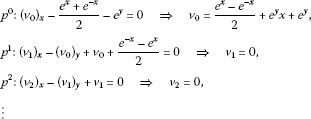Hence, the solution $u\left(x,y\right)$ becomes

$u\left(x,y\right)={v}_{0}=\frac{{e}^{x}-{e}^{-x}}{2}+{e}^{y}x+{e}^{y},$
(37)

which is the exact solution of the problem with minimum amount of calculation.

### 5.2 Example 2

Consider the following inhomogeneous linear BVP with variable coefficients:

$\begin{array}{r}{u}_{x}+y{u}_{y}-u=2{y}^{2}+2x{y}^{2},\\ u\left(0,y\right)=0.\end{array}$
(38)

In this problem, the right-hand side function$f\left(x,y\right)$ is a third-order polynomial. From (15), we have

$f\left(x,y\right)={f}_{1}\left(x,y\right)+y{S}_{y}\left({f}_{1}\left(x,y\right)\right)-S\left({f}_{1}\left(x,y\right)\right).$
(39)

Based on the source function $f\left(x,y\right)=2{y}^{2}+2x{y}^{2}$, if we take ${f}_{1}\left(x,y\right)$ as

${f}_{1}\left(x,y\right)={a}_{1}{x}^{3}+{a}_{2}{x}^{2}y+{a}_{3}x{y}^{2}+{a}_{4}{y}^{3}+{a}_{5}{x}^{2}+{a}_{6}xy+{a}_{7}{y}^{2}+{a}_{8}x+{a}_{9}y+{a}_{0}$
(40)

and substitute (40) into (39), we obtain

$\begin{array}{rcl}{a}_{7}& =& 2,\\ {a}_{1}& =& {a}_{2}={a}_{3}={a}_{4}={a}_{5}={a}_{6}={a}_{8}={a}_{9}={a}_{0}=0.\end{array}$

Based on these results, we have ${f}_{1}\left(x,y\right)=2{y}^{2}$, ${f}_{2}\left(x,y\right)=2x{y}^{2}$ and the homotopy is constructed in the following form:

$H\left(v,p\right)={v}_{x}-2{y}^{2}+p\left(y{v}_{y}-v-2x{y}^{2}\right)=0,$
(41)

and we get the following: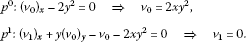Hence, we obtain the exact solution in the following form:

$u\left(x,y\right)={v}_{0}=2x{y}^{2}$
(42)

with short-length calculation.

### 5.3 Example 3

Let us find the solution of following BVP:

$\begin{array}{r}{u}_{x}+{u}_{y}-xu=y{e}^{xy}+x,\\ u\left(0,y\right)=0.\end{array}$
(43)

As in the previous examples, using (15) we get

$y{e}^{xy}+x={f}_{1}+{S}_{y}\left({f}_{1}\right)-xS\left({f}_{1}\right).$
(44)

Based on the source function $f\left(x,y\right)=y{e}^{xy}+x$, we conclude that ${f}_{1}\left(x,y\right)$ is in the following form:

${f}_{1}\left(x,y\right)={a}_{1}{e}^{xy}+{a}_{2}x{e}^{xy}+{a}_{3}x.$
(45)

Substituting (45) into (44), we obtain

$\begin{array}{rcl}{a}_{1}& =& {a}_{2}=0,\\ {a}_{3}& =& 1.\end{array}$

Now, these results let us take ${f}_{1}\left(x,y\right)=y{e}^{xy}$, ${f}_{2}\left(x,y\right)=x$ and construct the homotopy in the following form:

$H\left(v,p\right)={v}_{x}-y{e}^{xy}+p\left({v}_{y}-xv-x\right)=0,$
(46)

which leads to the following equations: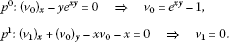Hence, the exact solution becomes

$u\left(x,y\right)={v}_{0}={e}^{xy}-1,$
(47)

which is obtained by minimum amount of calculations.

### 5.4 Example 4

Let us find the solution of the following first-order non-linear BVP:

$\begin{array}{r}{u}_{x}+{u}_{y}-{u}^{2}=-{e}^{2x}+3{e}^{x}-1,\\ u\left(0,y\right)=0.\end{array}$
(48)

Using (15) we get

$-{e}^{2x}+3{e}^{x}-1={f}_{1}+{S}_{y}\left({f}_{1}\right)-{\left[S\left({f}_{1}\right)\right]}^{2}.$
(49)

Based on the source function $f\left(x,y\right)=-{e}^{2x}+3{e}^{x}-1$, we find ${f}_{1}\left(x,y\right)$ is in the following form:

${f}_{1}\left(x,y\right)={a}_{1}{e}^{x}+{a}_{2}{e}^{2x}+{a}_{3}.$
(50)

Substituting (50) into (49), we obtain

$\begin{array}{rcl}{a}_{1}& =& 1,\\ {a}_{2}& =& {a}_{3}=0.\end{array}$

Now we can take ${f}_{1}\left(x,y\right)={e}^{x}$, ${f}_{2}\left(x,y\right)=-{e}^{2x}+2{e}^{x}-1$ and construct the homotopy in the following form:

$H\left(v,p\right)={v}_{x}-{e}^{x}+p\left({v}_{y}-{v}^{2}+{e}^{2x}-2{e}^{x}+1\right)=0,$
(51)

which leads to the following equations: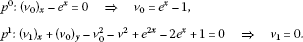Hence, we obtain the exact solution

$u\left(x,y\right)={v}_{0}={e}^{x}-1$
(52)

with short-length calculation.

## 6 Conclusion

In this paper, we employ a new homotopy perturbation method to obtain the solution of a first-order inhomogeneous PDE. In this method, each decomposition of the source function $f\left(x,y\right)$ leads to a new homotopy. However, we develop a method to obtain the proper decomposition of $f\left(x,y\right)$ which lets us obtain the solution with minimum computation and accelerate the convergence of the solution. This study shows that the decomposition of the source function has a great effect on the amount of computations and the acceleration of the convergence of the solution. Comparing to the standard one, decomposing the source function $f\left(x,y\right)$ properly is a simple and very effective tool for calculating the exact or approximate solutions with less computational work.

## References

1. Biazar J, Azimi F: He’s homotopy perturbation method for solving Helmoltz equation. Int. J. Contemp. Math. Sci. 2008, 3: 739-744.

2. Biazar J, Ghazvini H: Convergence of the homotopy perturbation method for partial differential equations. Nonlinear Anal., Real World Appl. 2009, 10: 2633-2640. 10.1016/j.nonrwa.2008.07.002

3. Sweilam NH, Khader MM: Exact solutions of some coupled nonlinear partial differential equations using the homotopy perturbation method. Comput. Math. Appl. 2009, 58: 2134-2141. 10.1016/j.camwa.2009.03.059

4. Biazar J, Ghazvini H: Homotopy perturbation method for solving hyperbolic partial differential equations. Comput. Math. Appl. 2008, 56: 453-458. 10.1016/j.camwa.2007.10.032

5. Junfeng L: An analytical approach to the sine-Gordon equation using the modified homotopy perturbation method. Comput. Math. Appl. 2009, 58: 2313-2319. 10.1016/j.camwa.2009.03.071

6. Neamaty A, Darzi R: Comparison between the variational iteration method and the homotopy perturbation method for the Sturm-Liouville differential equation. Bound. Value Probl. 2010., 2010: Article ID 317369

7. He JH: Homotopy perturbation technique. Comput. Methods Appl. Mech. Eng. 1999, 178: 257-262. 10.1016/S0045-7825(99)00018-3

8. He JH: A coupling method of homotopy technique and a perturbation technique for non-linear problems. Int. J. Non-Linear Mech. 2000, 35: 37-43. 10.1016/S0020-7462(98)00085-7

9. He JH: Homotopy perturbation method: a new nonlinear analytical technique. Appl. Math. Comput. 2003, 135: 73-79. 10.1016/S0096-3003(01)00312-5

10. He JH: Comparison of homotopy method and homotopy analysis method. Appl. Math. Comput. 2004, 156: 527-539. 10.1016/j.amc.2003.08.008

## Acknowledgements

Dedicated to Professor Hari M Srivastava.

The research was supported by parts by the Scientific and Technical Research Council of Turkey (TUBITAK).

## Author information

Authors

### Corresponding author

Correspondence to Ali Demir.

### Competing interests

The authors declare that they have no competing interests.

### Authors’ contributions

The authors declare that the study was realized in collaboration with the same responsibility. All authors read, checked and approved the final manuscript.

## Rights and permissions

Reprints and Permissions

Demir, A., Erman, S., Özgür, B. et al. Analysis of the new homotopy perturbation method for linear and nonlinear problems. Bound Value Probl 2013, 61 (2013). https://doi.org/10.1186/1687-2770-2013-61

• Accepted:

• Published:

• DOI: https://doi.org/10.1186/1687-2770-2013-61

### Keywords

• Exact Solution
• Boundary Value Problem
• Source Function
• Homotopy Analysis Method
• Computational Work